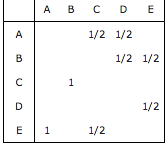### Google PageRank - Evaluating Simple Networks

I have been playing with the calculated PageRank for simple systems using the little web app I put together the other day.  This is partly to continue improving my understanding of the PageRank algorithm, but also to root out any issues with the implementation itself.

I was looking at one system and was concerned that the calculated solution was wrong.  This is because the end result was (and is) counter-intuitive.

Here is the basic little network:A "Simple" Network - Is It Obvious to You Why "C" should have a higher PageRank than "D"?
The circles correspond to web pages, and the links correspond to hyperlinks.  Here's the Google Matrix when the damping factor is one (this simplifies the numbers, but note that the ranking itself seems to be the same if you use a smaller damping factor):Google Matrix for System Above when Damping Factor is One

If we denote the entries of the PageRank vector x by [x_A, x_B, x_C, x_D, x_E], then based on the Google Matrix G above, the fact that the PageRank vector must satisfy x = Gx means that we should have

• x_A = (x_C + x_D)/2
• x_B = (x_D + x_E)/2
• x_C = x_B
• x_D = x_E/2
• x_E = x_A + x_C/2
Here's the calculated PageRank (scaled so that the values are integers):
• A: 15
• B: 18
• C: 18
• D: 12
• E: 24

This is all just arithmetic, written out here as part of confirming the answer once again.

The bigger issue to me is how to explain the result in any kind of simple or intuitive manner.  Could you have predicted that Page D would have a PageRank less than page C, especially if I told you that E was going to have a higher PageRank than B?  Page D seems to get ALL of Page E's PageRank, which has the most PageRank of any of the pages.  Page C gets all of B's PageRank, but Page B has less than Page E.  The results are not intuitive.

And this is only a web of five pages.  What does this say about trying to reliably predict the impact of adding/removing links when there are 42 billion nodes.  Where, if each page is represented by a standard marble, the array of marbles would not just stretch to 70% of the distance to the moon - which is the case when each column takes up about a quarter inch on your screen - but past it?   Where the removal of a single link somewhere might tilt equilibrium and shift page rankings worth billions of dollars.  This amazing performance by Miyoki Shida Rigolo comes to mind:

The Impact of Little Things in a Connected World

This being a bit overly dramatic, I realize.  And I understand that PageRank (or rather, its evolved form internal to Google) is only one of many factors used in determining the rankings.  Nevertheless, I still have experimentation and reading-up to do on this area in search of the "explanation for a five-year-old".

Please feel free to let me know what you find as well.  Perhaps something obvious is being overlooked here.